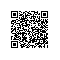# 查找 算法

1.顺序查找（不在讨论）

2.二分查找，插值查找，斐波那契查找

3.树表查找

4.分块查找

5.哈希查找

public function BinarySearch($a=array(),$val,$n){$low=0;

$high =$n-1;

$mid = 0; while($low<=$high){$mid = ($low+$high)/2;

if($a[$mid]===$value) return mid; if($a[mid] >$value)$high = $mid – 1; if($a[mid]<$value)$low = $mid+1 } return false; } 插值查找（基于二分查找，对于查找点可以改进） 基本思想：基于二分查找算法，将查找点的选择改进为自适应选择，可以提高查找效率。当然，差值查找也属于有序查找。 注：对于表长较大，而关键字分布又比较均匀的查找表来说，插值查找算法的平均性能比折半查找要好的多。反之，数组中如果分布非常不均匀，那么插值查找未必是很合适的选择。 复杂度分析：查找成功或者失败的时间复杂度均为O(log2(log2n))。 public function InsertSearch($a=array(),$value,$low,$high){$mid = $low+($value-$a[low])/($a[$high]-$a[low])*($high-$low);

if($a[$mid]===$value) return mid; if($a[mid] >$value) InsertSearch($a,$value,$low,$mid-1); if($a[mid]<=$value) InsertSearch($a,$value,$mid+1,$high); } ##### 斐波那契查找 基本思想：也是二分查找的一种提升算法，通过运用黄金比例的概念在数列中选择查找点进行查找，提高查找效率。同样地，斐波那契查找也属于一种有序查找算法。 构建斐波那契数组 private$max_size = 20;

private function Fibonacci(){

$F = 1;$F = 1;

for($i =2;$i<$this->max_size;==i){$F[$i] =$[$-1]+$F[$i-2]; } return$F;

}

public function FibonacciSearch($a=array(),$n,$key){$low = 0;

$high =$n-1;

$F=$this->Fibonacci();

$k = 0; while(n>F[k]-1) ++k; //将数组a扩展到F[k]-1的长度$temp;

for($i =$n;$i<F[k]-1;++i){$temp[$i] =$a[$n-1]; } while($low<=$high){$mid = $low + F[k-1] –1; if($key<$temp[$mid]){

$high =$mid-1;

$k-=1; } else if($key>$temp[$mid]){

$low =$mid+1;

$k-=2; }else{ if($mid<n)

return $mid; else return n-1; } } unset($temp);

}

B树和B+树

B树可以看作是对2-3查找树的一种扩展，即他允许每个节点有M-1个子节点。

B+树是对B树的一种变形树，它与B树的差异在于：

• 有k个子结点的结点必然有k个关键码；
• 非叶结点仅具有索引作用，跟记录有关的信息均存放在叶结点中。
• 树的所有叶结点构成一个有序链表，可以按照关键码排序的次序遍历全部记录

step1 先选取各块中的最大关键字构成一个索引表；
step2 查找分两个部分：先对索引表进行二分查找或顺序查找，以确定待查记录在哪一块中；然后，在已确定的块中用顺序法进行查找。

哈希函数的规则是：通过某种转换关系，使关键字适度的分散到指定大小的的顺序结构中，越分散，则以后查找的时间复杂度越小，空间复杂度越高。

Hash是一种典型以空间换时间的算法，比如原来一个长度为100的数组，对其查找，只需要遍历且匹配相应记录即可，从空间复杂度上来看，假如数组存储的是byte类型数据，那么该数组占用100byte空间。现在我们采用Hash算法，我们前面说的Hash必须有一个规则，约束键与存储位置的关系，那么就需要一个固定长度的hash表，此时，仍然是100byte的数组，假设我们需要的100byte用来记录键与位置的关系，那么总的空间为200byte,而且用于记录规则的表大小会根据规则，大小可能是不定的。使用钉钉扫一扫加入圈子
+ 订阅